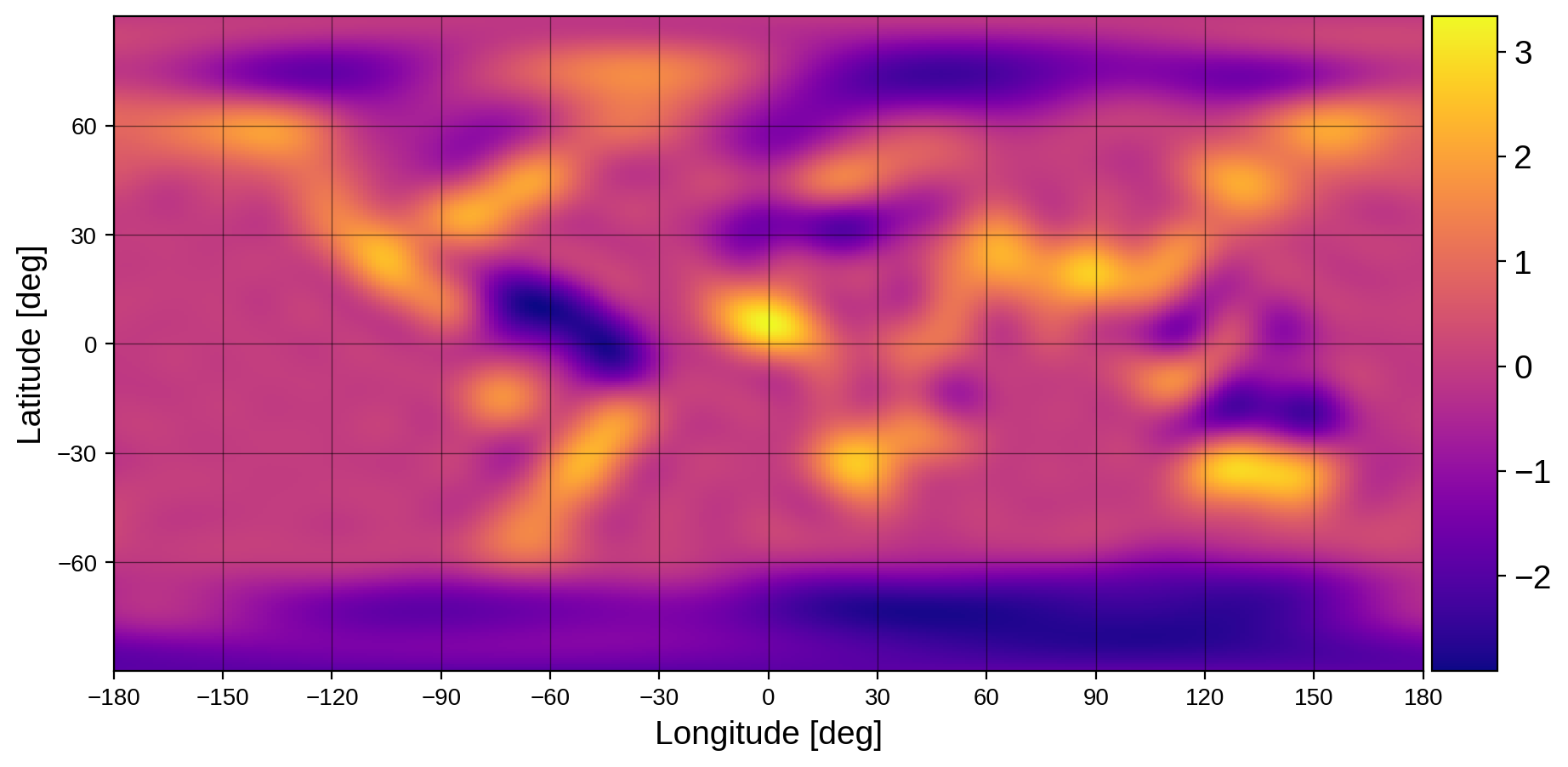Note

This tutorial was generated from a Jupyter notebook that can be downloaded here.

# The pixel transform¶

Even though it is convenient to define surface maps in the spherical harmonic basis (because it allows us to compute fluxes analytically), the spherical harmonic basis does have some downsides. The main one relates to the fact that it’s really hard to ensure positivity of a surface map when we’re in the $$Y_{lm}$$ basis. Since spherical harmonics are polynomials on the surface of the sphere, ensuring positivity of a degree $$l$$ spherical harmonic expansion is equivalent to ensuring a polynomial of the same total degree has no roots, which isn’t trivial.

It’s much easier to ensure positivity in pixel space, i.e., on a discrete grid on the surface of the sphere. This notebook discusses how to use the get_pixel_tranforms method to obtain the linear operators that transform back and forth between pixels (on a Mollweide grid) and spherical harmonics.

:

import starry
import matplotlib.pyplot as plt

starry.config.lazy = False
starry.config.quiet = True


We begin by instantiating a map of the Earth at $$l = 20$$:

:

map = starry.Map(20)
y0 = np.array(map.y)
fig, ax = plt.subplots(1, figsize=(12, 5))
map.show(ax=ax, projection="rect", colorbar=True)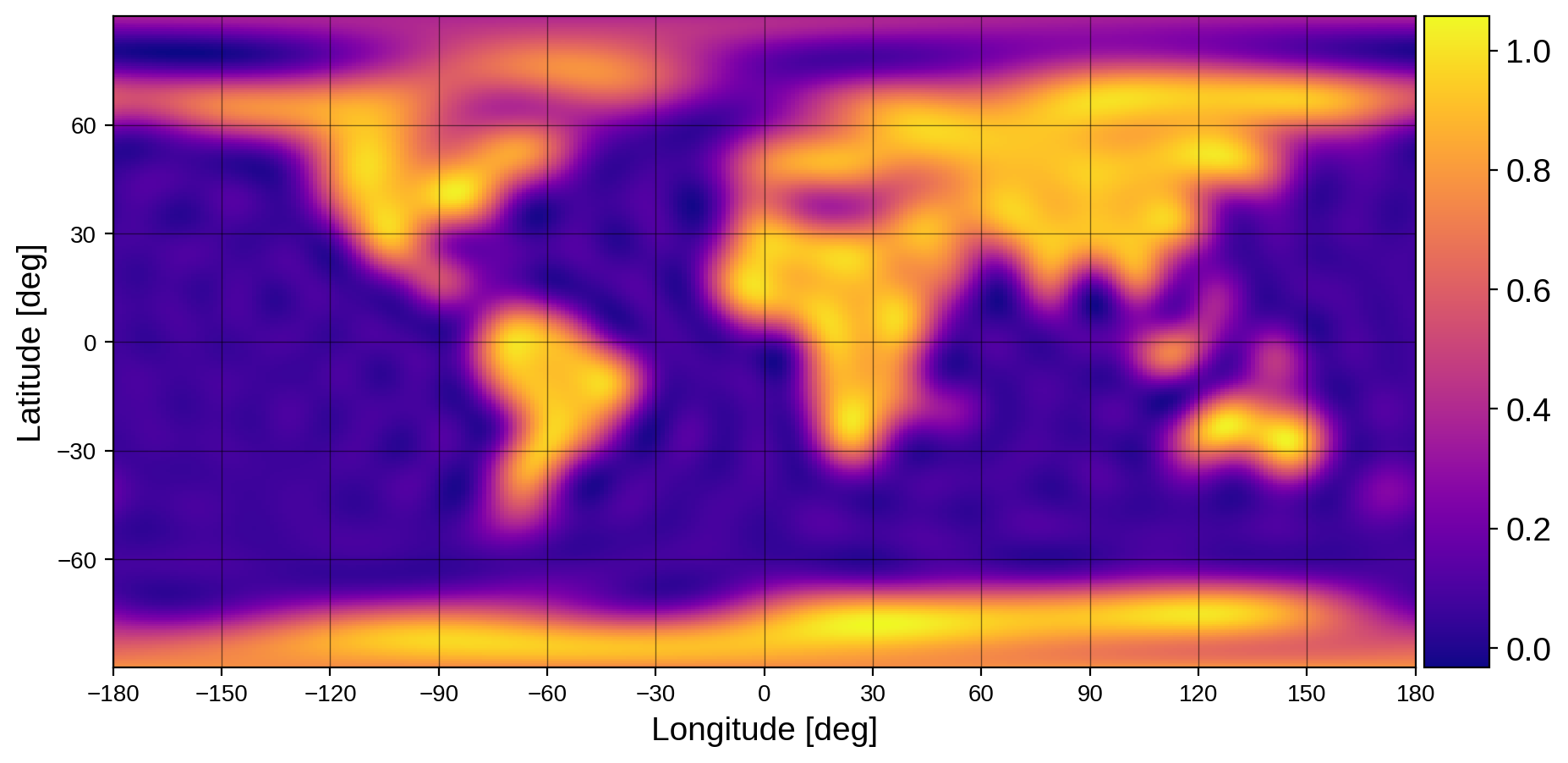Now let’s get the pixel transform on a Mollweide grid:

:

lat, lon, Y2P, P2Y, Dx, Dy = map.get_pixel_transforms()


Here are the two matrices that transform spherical harmonics to pixels and pixels to spherical harmonics, respectively:

:

fig, ax = plt.subplots(1, 2, figsize=(15, 7))
ax.imshow(np.log10(np.abs(Y2P)), vmin=-10)
ax.imshow(np.log10(np.abs(P2Y)), vmin=-10)
ax.set(xticks=[], yticks=[], xlabel=r"$N_{ylm}$", ylabel=r"$N_{pix}$", title="Y2P")
ax.set(xticks=[], yticks=[], xlabel=r"$N_{pix}$", ylabel=r"$N_{ylm}$", title="P2Y");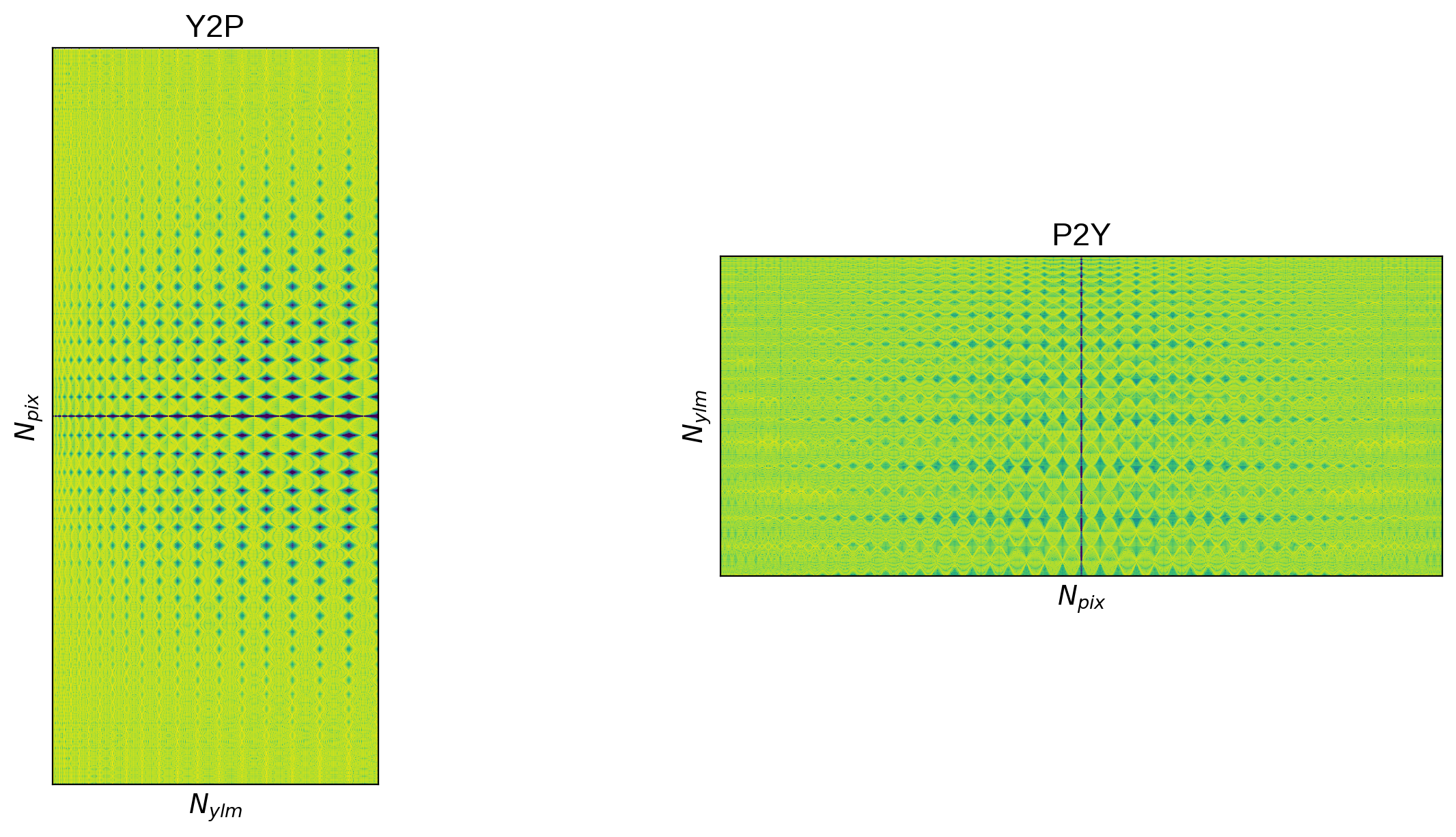Let’s get the pixel representation of our map…

:

p = Y2P.dot(map.y)


… and visualize this vector on a rectangular lat/lon grid:

:

fig, ax = plt.subplots(1, figsize=(12, 5))
im = ax.scatter(lon, lat, s=300, c=p, alpha=0.5, ec="none", cmap="plasma")
plt.colorbar(im)
ax.set_xlim(-190, 190)
ax.set_ylim(-90, 90)
ax.set_xlabel("Longitude [deg]")
ax.set_ylabel("Latitude [deg]");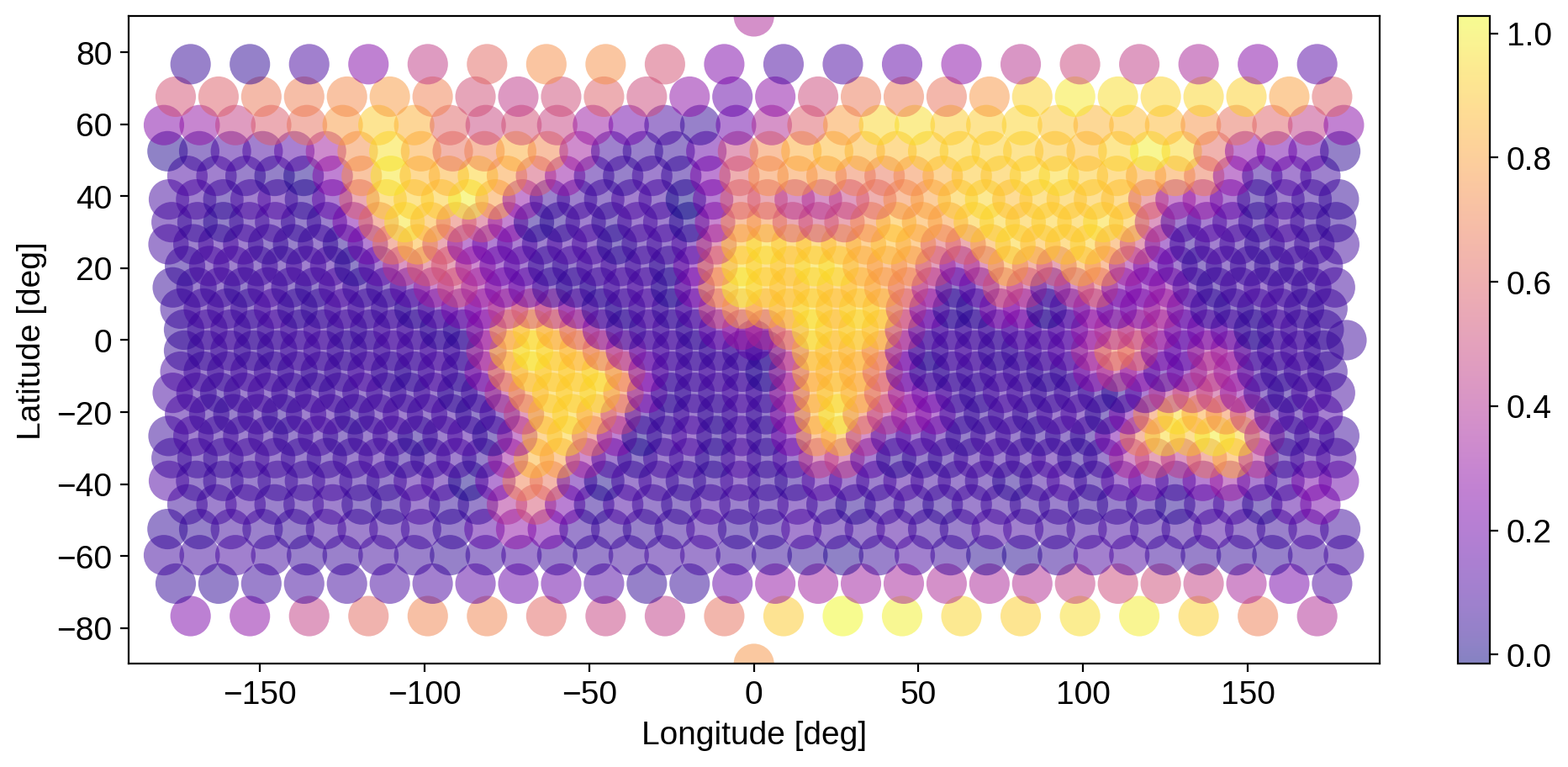That’s the forward transform. We can now transform back to spherical harmonics and see what we get:

:

y = P2Y.dot(p)


Here’s the difference between the original spherical harmonic vector and the vector after the full cycle of transformations. Because of numerics (and a small regularization term in the inversion), the transform isn’t exactyl one-to-one, but it’s close.

:

plt.plot(np.abs((y0 - y) / y0))
plt.yscale("log")
plt.ylabel("difference")
plt.xlabel("spherical harmonic index");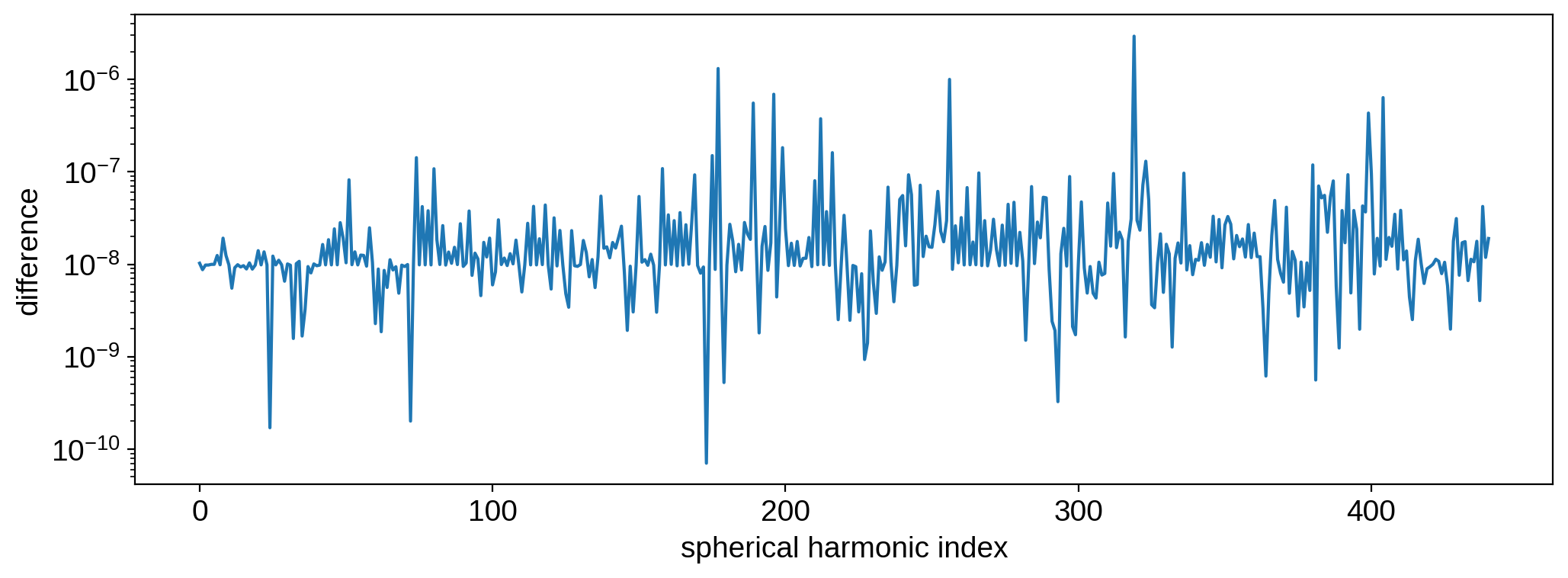We can also plot the new map using starry:

Note

Maps in starry require the coefficient of the $$Y_{0,0}$$ term to be unity. In order to ingest the new $$Y_{lm}$$ coefficients into starry, we divide them by the $$Y_{0,0}$$ term then set the map amplitude equal to the $$Y_{0,0}$$ term to get the correct scaling.

:

map[1:, :] = y[1:] / y
map.amp = y
fig, ax = plt.subplots(1, figsize=(12, 5))
map.show(ax=ax, projection="rect", colorbar=True)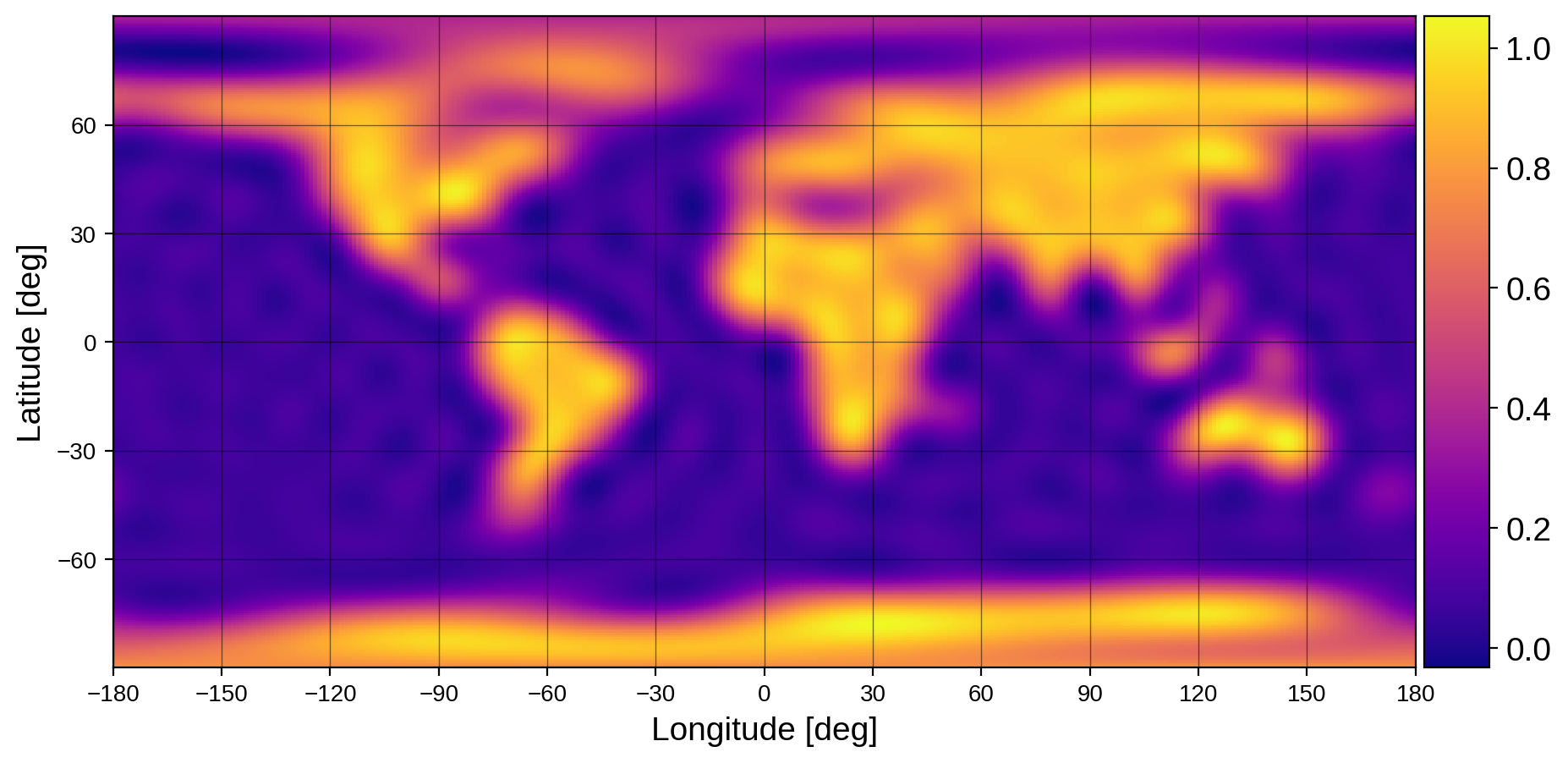## Differentiation¶

We can also obtain the derivatives of the pixel representation with respect to longitude and latitude via a purely linear operation:

:

# longitude derivative
Dxp = Dx.dot(p)
Dxp_y = P2Y.dot(Dxp)

map[1:, :] = Dxp_y[1:] / Dxp_y
map.amp = Dxp_y
fig, ax = plt.subplots(1, figsize=(12, 5))
map.show(ax=ax, projection="rect", colorbar=True)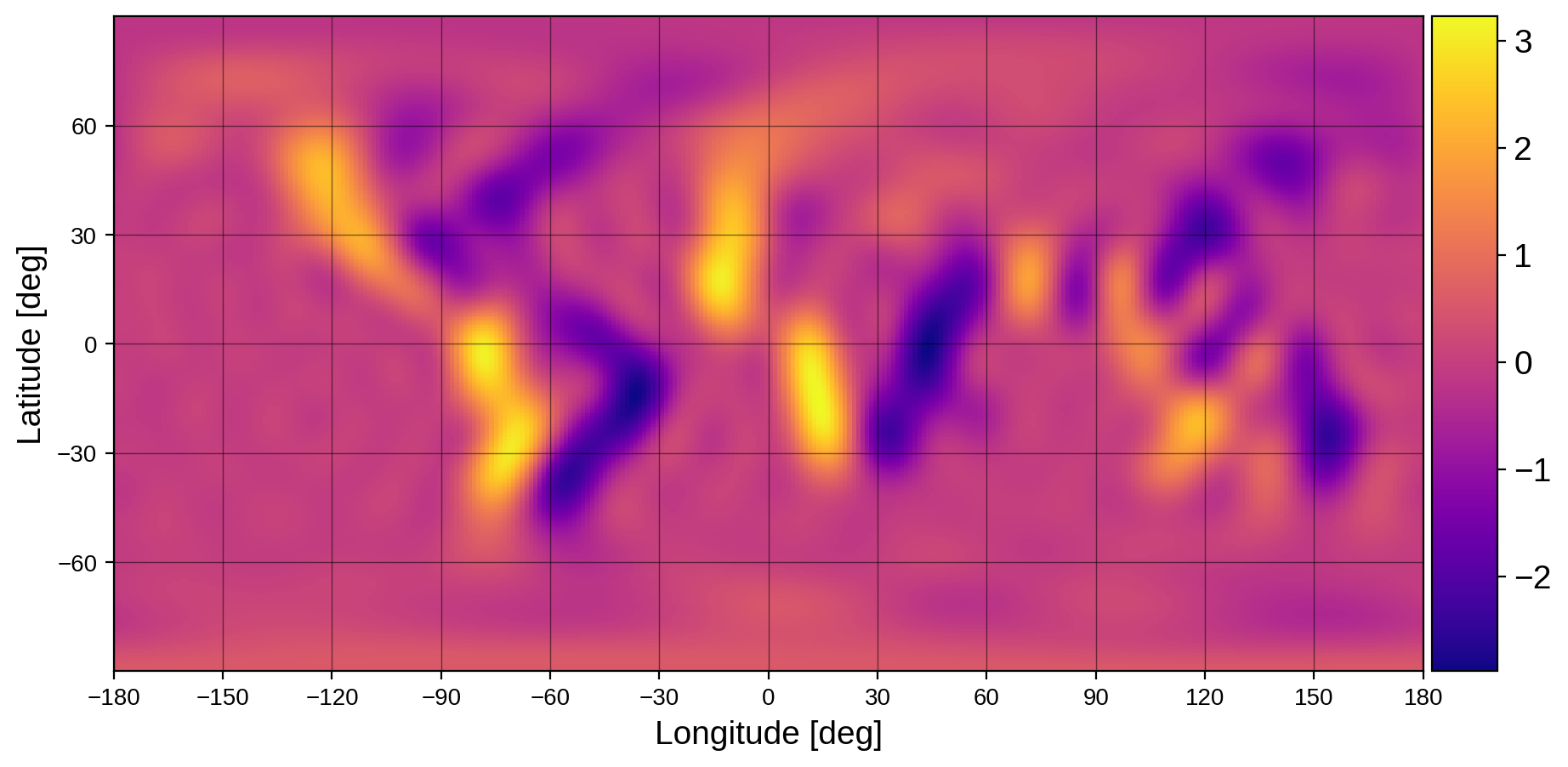:

# latitude derivative
Dyp = Dy.dot(p)
Dyp_y = P2Y.dot(Dyp)

map[1:, :] = Dyp_y[1:] / Dyp_y
map.amp = Dyp_y
fig, ax = plt.subplots(1, figsize=(12, 5))
map.show(ax=ax, projection="rect", colorbar=True)